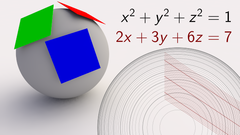## Massively Multivariable Open Online Calculus Course

 所在平台: Coursera 课程类别: 计算机科学Explore 1600+ online courses from top universities. Join Coursera today to learn data science, programming, business strategy, and more.#### 课程详情

M2O2C2 is an invitation to think carefully about how one thing changing affects something else. What is the "derivative" of a function of many variables? How can a curved object be approximated by a flat plane? What does the chain rule look like when many things are affecting many other things? How do we find an input which maximizes a function of many variables?

This is a first course in multivariable differential calculus, but along the way, we will also introduce a ton of linear algebra. The result is a course targeted at a student who has seen a bit of calculus and who is willing to learn about linear transformations and vectors; such a student will have the best possible vantage point from which to explore derivatives of functions of many variables.

#### 课程大纲

This course is a first taste of multivariable calculus, but viewed through the lens of linear algebra. Introducing matrices (and linear transformations!) permits us to share a more coherent story about multivariable differential calculus.

Week 1: Multiple variables
Week 2: Limits and derivatives
Week 3: The chain rule
Week 4: Second derivative
Week 5: Optimization
Week 6: Higher derivatives

#### 欢迎关注我们的公众号#### 课程简介

M2O2C2 provides a first taste of multivariable differential calculus. By introducing the machinery of linear algebra, this course provides helpful tools for understanding the derivative of a function of many variables.

#### 课程标签

M2O2C2 多元微积分 多变量微积分

#### 1人关注该课程